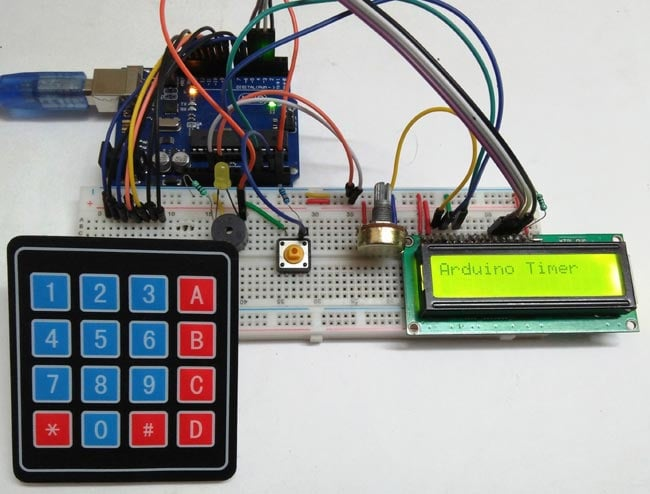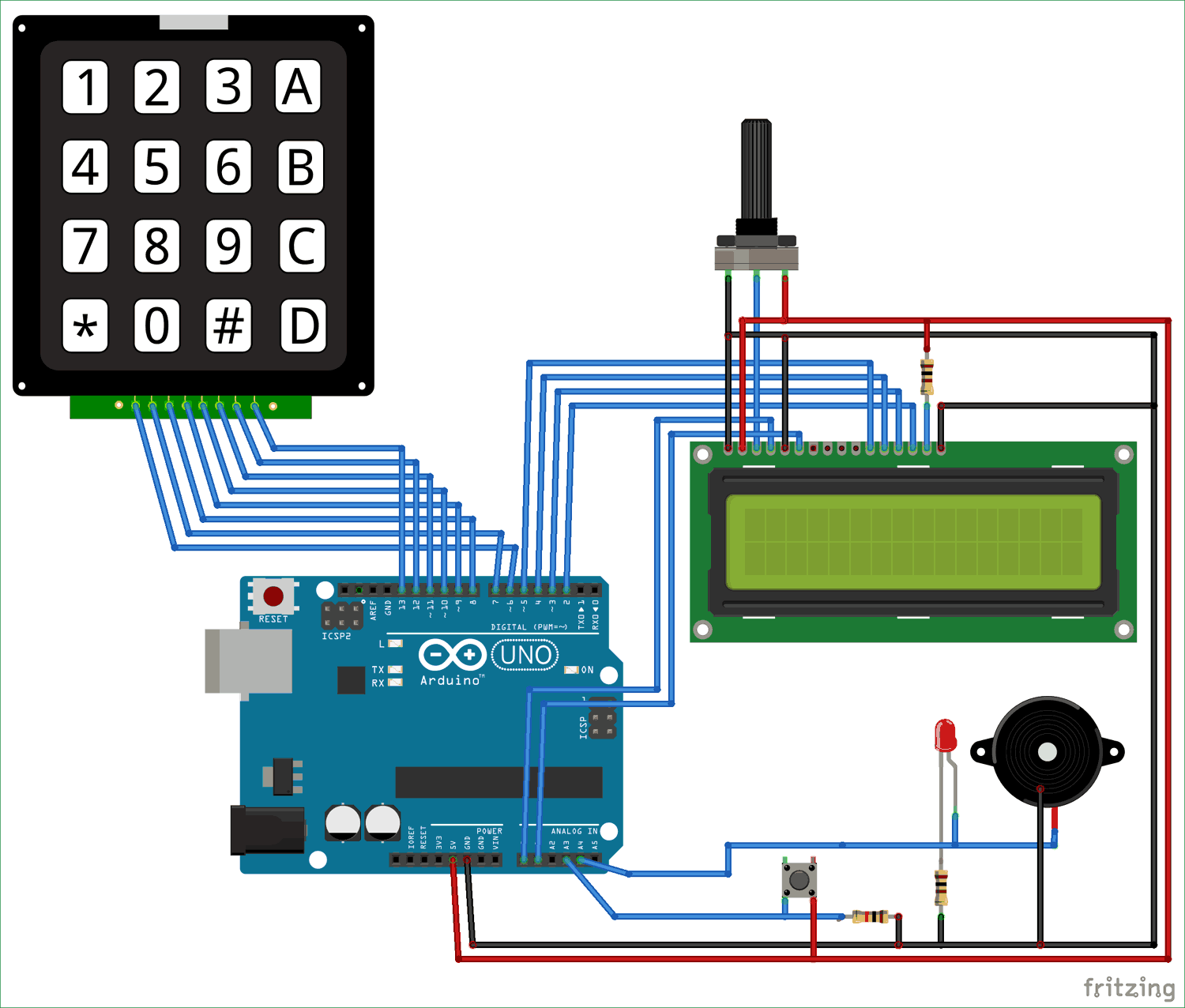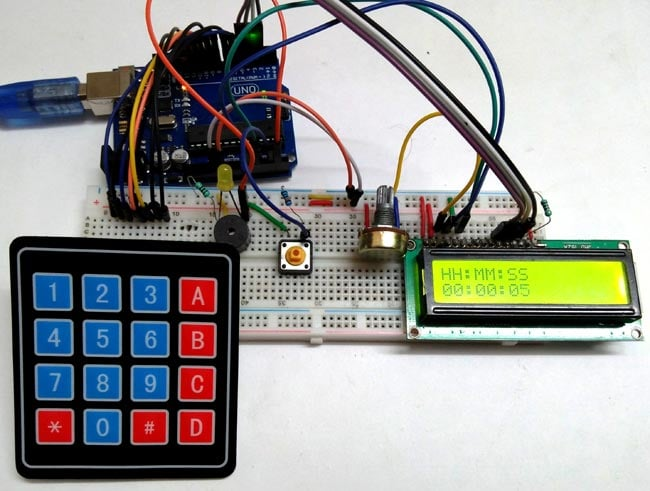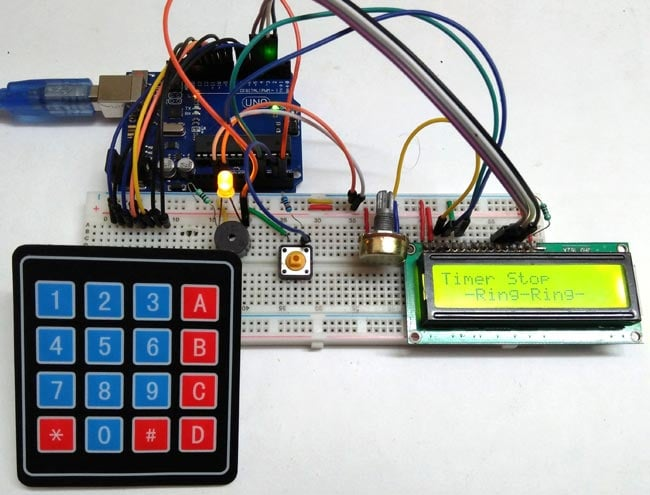# Arduino Countdown Timer

Published  April 17, 2018   12A timer is a type of clock used for the measurement of time intervals. There are two types of timer, one which counts upwards from zero, for the measurement of the elapsed time, called as Stopwatch. And, the second one counts down from a specified time duration provided by the user, generally called as Countdown Timer.

Here, in this tutorial we will show you how to make a Countdown Timer using Arduino. Here we are not using any Real Time Clock (RTC) module for getting the time. The time duration is provided by the user with the help of Keypad and 16x2 LCD. And when the timer reaches to Zero, alert sound will be produced with the help of Buzzer.

### Material Required

• Arduino UNO
• LCD 16*2
• Buzzer
• Pushbutton
• Potentiometer (10k)
• Resistor (10k, 100 ohm)
• Connecting wires

### Arduino Countdown Timer Circuit DiagramArduino Uno is used here as main controller. A keypad is used for feeding the time duration and a 16*2 LCD is used to display the countdown. The pushbutton is used to start the time. Check here how to interface 4x4 Keypad with Arduino and 16x2 LCD with Arduino.

### Arduino Countdown Timer Code and Explanation

Complete Arduino Timer code is given at the end of this Project.

In this code below, we are initializing libraries for keypad and LCD and the variables used in the code.

```#include <LiquidCrystal.h>

long int set1;
long int set2;
long int set3;
long int set4;
long int j;
int t1, t2, t3, t4, t5, t6;
int r1, r2, r3;
char key;
String r;
String hours;
String minutes;
String seconds;
```

Now, in the below code we are initializing the no. of rows and columns for defining the matrix for keypad.

```const byte ROWS = 4; // Four rows
const byte COLS = 4; // Three columns

char keys[ROWS][COLS] = {
{'1','2','3','A'},
{'4','5','6','B'},
{'7','8','9','C'},
{'*','0','#','D'}
};```

For connecting the 4*4 matrix keypad with Arduino we have to define the pins for the rows and columns. So in below code we have defined pins for Keypad as well as 16x2 LCD.

```byte rowPins[ROWS] = { 6, 7, 8, 9 };// Connect keypad ROW0, ROW1, ROW2 and ROW3 to these Arduino pins
byte colPins[COLS] = { 10, 11, 12, 13 };// Connect keypad COL0, COL1 and COL2 to t

LiquidCrystal lcd(A0, A1, 5, 4, 3, 2); // Creates an LC object. Parameters: (rs, enable, d4, d5, d6, d7)```

The below code is used for making the keypad,

`Keypad kpd = Keypad( makeKeymap(keys), rowPins, colPins, ROWS, COLS );`

In the void setFeedingTime() function code, after pressing the pushbutton we are able to enter the time for timer, then after entering the timer time duration, we have to Press D to begin the countdown.

``` void setFeedingTime()
{
feed = true;
int i=0;

lcd.clear();
lcd.setCursor(0,0);
lcd.print("Set feeding Time");
lcd.clear();
lcd.print("HH:MM:SS");
lcd.setCursor(0,1);

while(1){
key = kpd.getKey();
char j;

if(key!=NO_KEY){
lcd.setCursor(j,1);
lcd.print(key);
r[i] = key-48;
i++;
j++;

if (j==2 || j == 5)
{
lcd.print(":"); j++;
}
delay(500);
}

if (key == 'D')
{key=0; break; }
}
lcd.clear();
}```

In void setup() function, we have initialized the LCD and serial communication, and defined the pins as INPUT and OUTPUT in the below code.

``` void setup()
{
lcd.begin(16,2);
Serial.begin(9600);
pinMode(A0, OUTPUT);
pinMode(A1, OUTPUT);
pinMode(A3, INPUT);
pinMode(A4, OUTPUT);
}```

Working of this Arduino Countdown Timer is simple but the code is a little complex. The code is explained by the comments in the code.

Initially, it will print “Arduino Timer” on the LCD display until you press the pushbutton. As soon as you press the pushbutton, it will ask to enter countdown time duration by calling the “setFeedingTime” function. Then you can enter the time duration with the help of Keypad. Then you need to press ‘D’ to save the time and begin the countdown timer. Here in void loop() function, we have done some calculation to decrement the time second by second and to show the proper values of Hour, Minutes and Seconds (HH:MM:SS) according to the remaining time. All the code is well explained by comments. You can check the complete code and Demonstration video below.As the timer reaches to zero, the buzzer starts beeping and beeps for 100 times only (as per the code). To stop the buzzer, press and hold the pushbutton. You can use the Pushbutton anytime to stop the timer in between counting.Code

// Arduino Countdown Timer Code

#include <LiquidCrystal.h>

const byte ROWS = 4; // Four rows
const byte COLS = 4; // Three columns

long int set1;
long int set2;
long int set3;
long int set4;
long int j;

String hours;
String minutes;
String seconds;
// Define the Keymap

char keys[ROWS][COLS] = {

{'1','2','3','A'},

{'4','5','6','B'},

{'7','8','9','C'},

{'*','0','#','D'}

};

byte rowPins[ROWS] = { 6, 7, 8, 9 };// Connect keypad ROW0, ROW1, ROW2 and ROW3 to these Arduino pins
byte colPins[COLS] = { 10, 11, 12, 13 };// Connect keypad COL0, COL1 and COL2 to t

LiquidCrystal lcd(A0, A1, 5, 4, 3, 2); // Creates an LC object. Parameters: (rs, enable, d4, d5, d6, d7)

int t1, t2, t3, t4, t5, t6;
int r1, r2, r3;

boolean feed = true; // condition for alarm

char key;
String r;

void setFeedingTime()
{
feed = true;
int i=0;

lcd.clear();
lcd.setCursor(0,0);
lcd.print("Set feeding Time");
lcd.clear();
lcd.print("HH:MM:SS");
lcd.setCursor(0,1);

while(1){
key = kpd.getKey();

char j;

if(key!=NO_KEY){

lcd.setCursor(j,1);

lcd.print(key);

r[i] = key-48;
i++;
j++;

if (j==2 || j == 5)
{
lcd.print(":"); j++;
}
delay(500);
}

if (key == 'D')
{key=0; break; }
}
lcd.clear();
}

void setup()
{
lcd.begin(16,2);
Serial.begin(9600);
pinMode(A0, OUTPUT);
pinMode(A1, OUTPUT);
pinMode(A3, INPUT);
pinMode(A4, OUTPUT);
}

void loop()
{
lcd.setCursor(0,0);
lcd.print("Arduino Timer");
//Serial.println(A3);

{
lcd.clear();
setFeedingTime();
for(int i = 0; i < 6; i++)  // this for loop is used to get the value of the feeding time and print it serially
{
Serial.print(r[i]);
Serial.println();
}

hours = String (r) + String (r) ; //combining two separate int values of r and r into one string and save it to "hours"
minutes = String (r) + String (r) ;  //combining two separate int values of r and r into one string and save it to "minutes"
seconds = String (r) + String (r) ;  //combining two separate int values of r and r into one string and save it to "seconds"

set1 = (hours.toInt()*3600); //converting hours into seconds
set2 = (minutes.toInt() * 60);  //converting minutes into seconds
set3 = seconds.toInt();
set4 = (hours.toInt() * 3600)+ (minutes.toInt() * 60) + seconds.toInt(); //adding set1, set2 and set3 together in set4
Serial.print("set4");
Serial.print(set4);
Serial.println();

lcd.setCursor(0,0);
lcd.print("Countdown begins");
delay(1000);
lcd.clear();

for(long int j = set4; j >= 0; j--) // this for loopis used to decrease the total time in seconds
{
Serial.println(j);

lcd.setCursor(0,0);
lcd.print("HH:MM:SS");

long int HH = j / 3600; // converting the remaining time into remaining hours
lcd.setCursor(0,1);
Serial.println(HH);
if (HH < 10) { lcd.print('0');  }
lcd.print(HH);
lcd.print(":");

long int MM = (j - (HH*3600))/60 ; //converting the remaining time into remaining minutes
lcd.setCursor(3,1);
Serial.println(MM);
if (MM < 10) { lcd.print('0');  }
lcd.print(MM);
lcd.print(":");

long int SS = j - ((HH*3600)+(MM*60)); //converting the remaining time into remaining seconds
lcd.setCursor(6,1);
Serial.println(SS);
if (SS < 10) { lcd.print('0');  }
lcd.print(SS);
delay(1000);

if (j == 0)

{
lcd.clear();
lcd.setCursor(0,0);
lcd.print("Timer Stop");
lcd.setCursor(2,1);
lcd.print("-Ring-Ring-");

for(int k =0; k<= 100; k++) //this for loop is used for the buzzer to beep 100 time as the timer reaches zero
{
digitalWrite(A4,HIGH);
delay(300);
digitalWrite(A4,LOW);
delay(300);
}
}
}
}
}

Tags

Submitted by Gondola karan on Wed, 04/18/2018 - 18:08

### It's const byte COLS = 4; //

It's const byte COLS = 4; // four columns

Submitted by mohamed on Sat, 04/21/2018 - 01:50

### thank u

thank u good project

Submitted by SRQPoppi on Sun, 06/24/2018 - 02:01

Thanks....

Submitted by Silas on Sat, 07/07/2018 - 22:04

### I really like this project

I really like this project but for some reason I can´t put the code into my arduino

Submitted by Larry on Thu, 08/30/2018 - 14:07

### Me too and error appear below

Me too and error appear below.

"Error compiling for board Arduino/Genuino Uno"

### count down timer

Very nice project , was OK at the first time . I use it for my PCB printing machine . Keep up the good work.

F8VOA

### I need a 6 month countdown

I need a 6 month countdown timer to act as a 'service indicator'. Can this project be amended to either a) Add more hour values (i.e. HHHH:MM:SS - I would need 4320 hours by this means) or b) Add months and days values (i.e. MM:DD) as opposed to hours, minutes and seconds? Accuracy is not an issue, as we can afford quite a bit of leeway, but would there be any other issues in doing this? Could the unit handle a 15,552,000 (60 seconds x 60 minutes x 24 hours x 180 days) seconds countdown? Quite new to all this and looking for ideas on a project I have been asked to look at.

### Help!!!

I tried to add days to this timer, but I don't know why it doesn't work

#include <LiquidCrystal.h>

const byte ROWS = 4; // Four rows
const byte COLS = 4; // Three columns

long int set1;
long int set2;
long int set3;
long int set4;
long int j;

String days;
String hours;
String minutes;
String seconds;
// Define the Keymap

char keys[ROWS][COLS] = {

{'1','2','3','A'},

{'4','5','6','B'},

{'7','8','9','C'},

{'*','0','#','D'}

};

byte rowPins[ROWS] = { 6, 7, 8, 9 };// Connect keypad ROW0, ROW1, ROW2 and ROW3 to these Arduino pins
byte colPins[COLS] = { 10, 11, 12, 13 };// Connect keypad COL0, COL1 and COL2 to t

LiquidCrystal lcd(A0, A1, 5, 4, 3, 2); // Creates an LC object. Parameters: (rs, enable, d4, d5, d6, d7)

int t1, t2, t3, t4, t5, t6;
int r1, r2, r3;

boolean feed = true; // condition for alarm

char key;
String r;

void setFeedingTime()
{
feed = true;
int i=0;

lcd.clear();
lcd.setCursor(0,0);
lcd.print("Nustatykite laika");
lcd.clear();
lcd.print("DD:VV:MM:SS");
lcd.setCursor(0,1);

while(1){
key = kpd.getKey();

char j;

if(key!=NO_KEY){

lcd.setCursor(j,1);

lcd.print(key);

r[i] = key-48;
i++;
j++;

if (j==2 || j == 5)
{
lcd.print(":"); j++;
}
delay(500);
}

if (key == 'D')
{key=0; break; }
}
lcd.clear();
}

void setup()
{
lcd.begin(16,2);
Serial.begin(9600);
pinMode(A0, OUTPUT);
pinMode(A1, OUTPUT);
pinMode(A3, INPUT);
pinMode(A4, OUTPUT);
}

void loop()
{
lcd.setCursor(0,0);
lcd.print("Kaledu laikrodis");
//Serial.println(A3);

{
lcd.clear();
setFeedingTime();
for(int i = 0; i < 6; i++)  // this for loop is used to get the value of the feeding time and print it serially
{
Serial.print(r[i]);
Serial.println();
}

days = String (r) + String (r) ; //combining two separate int values of r and r into one string and save it to "days"
hours =  String (r) + String (r) ;  //combining two separate int values of r and r into one string and save it to "hours"
minutes = String (r) + String (r) ;  //combining two separate int values of r and r into one string and save it to "minutes"
seconds =  String (r)+ String (r);  //combining two separate int values of r and r into one string and save it to "seconds"

set1 = (days.toInt()*86400); //converting days into seconds
set2 = (hours.toInt()*3600); //converting hours into seconds
set3 = (minutes.toInt() * 60);  //converting minutes into seconds
set4 = seconds.toInt();
set4 = (days.toInt() * 86400)+ (hours.toInt() * 3600)+ (minutes.toInt() * 60) + seconds.toInt();
Serial.print("set4");
Serial.print(set4);
Serial.println();

lcd.setCursor(0,0);
lcd.print("Laikas tiksi");
delay(1000);
lcd.clear();

for(long int j = set4; j >= 0; j--) // this for loopis used to decrease the total time in seconds
{
Serial.println(j);

lcd.setCursor(0,0);
lcd.print("DD:VV:MM:SS");

long int DD = j / 86400; // converting the remaining time into remaining days
lcd.setCursor(0,1);
Serial.println(DD);
if (DD < 10) { lcd.print('0');  }
lcd.print(DD);
lcd.print(":");

long int VV = (j - (DD*86400))/60 ; // converting the remaining time into remaining hours
lcd.setCursor(0,1);
Serial.println(VV);
if (VV < 10) { lcd.print('0');  }
lcd.print(VV);
lcd.print(":");

long int MM = (j - (DD*86400)+(VV*3600))/60 ; //converting the remaining time into remaining minutes
lcd.setCursor(3,1);
Serial.println(MM);
if (MM < 10) { lcd.print('0');  }
lcd.print(MM);
lcd.print(":");

long int SS = j - ((DD*86400)+(VV*3600)+(MM*60)); //converting the remaining time into remaining seconds
lcd.setCursor(6,1);
Serial.println(SS);
if (SS < 10) { lcd.print('0');  }
lcd.print(SS);
delay(1000);

if (j == 0)

{
lcd.clear();
lcd.setCursor(0,0);
lcd.print("Laikas baigesi");
lcd.setCursor(2,1);
lcd.print("Kaledos");

for(int k =0; k<= 100; k++) //this for loop is used for the buzzer to beep 100 time as the timer reaches zero
{
digitalWrite(A4,HIGH);
delay(300);
digitalWrite(A4,LOW);
delay(300);
}
}
}
}
}

### I modified the code to be

I modified the code to be better (less laggy on the button and keypad and second set time is positioned properly), wish this more suit to you.

// Arduino Countdown Timer Code

#include <LiquidCrystal.h>

const byte ROWS = 4; // Four rows
const byte COLS = 4; // Three columns

long int set1;
long int set2;
long int set3;
long int set4;
long int j;

String hours;
String minutes;
String seconds;
// Define the Keymap

char keys[ROWS][COLS] = {

{'7', '8', '9', '/'},

{'4', '5', '6', '*'},

{'1', '2', '3', '-'},

{'C', '0', '=', '+'}

};

byte rowPins[ROWS] = { 6, 7, 8, 9 };// Connect keypad ROW0, ROW1, ROW2 and ROW3 to these Arduino pins
byte colPins[COLS] = { 10, 11, 12, 13 };// Connect keypad COL0, COL1 and COL2 to t

LiquidCrystal lcd(A0, A1, 5, 4, 3, 2); // Creates an LC object. Parameters: (rs, enable, d4, d5, d6, d7)

int t1, t2, t3, t4, t5, t6;
int r1, r2, r3;

boolean feed = true; // condition for alarm

char key;
String r;

void setFeedingTime()

A:
feed = true;
int i = 0;

lcd.clear();
lcd.setCursor(0, 0);
lcd.print("Set feeding Time");
delay(1000);
lcd.clear();
lcd.print("HH:MM:SS");
lcd.setCursor(0, 1);
int x = 0;

while (1) {
key = kpd.getKey();

if (key != NO_KEY && (x != 2 || x != 5)) {

if (key == 'C')
{
if (x==0)
{
x=0;
}
else if (x>0)
{
x-=1;
i-=1;
if (x == 2 || x == 5)
{
x-=1;
}
lcd.setCursor(x, 1);
lcd.print(" ");
}
}

else if (key != 'C')
{

lcd.setCursor(x, 1);

lcd.print(key);

r[i] = key-48 ;
i++;
x++;

}
}

else if (x == 2 || x == 5)
{
lcd.print(":"); x++;
}

delay(50);

if (key == '=')
break;

{
goto A;
}
}
lcd.clear();
}

void setup()
{
lcd.begin(16, 2);
Serial.begin(9600);
Serial.println("Debug log");
pinMode(A0, OUTPUT);
pinMode(A1, OUTPUT);
pinMode(A3, INPUT);
pinMode(A4, OUTPUT);
}

void loop()
{
lcd.setCursor(0, 0);
lcd.print("Arduino Timer");
//Serial.println(A3);

{
lcd.clear();
setFeedingTime();
for (int i = 0; i < 6; i++) // this for loop is used to get the value of the feeding time and print it serially
{
Serial.print(r[i]);
Serial.print(" ");
}

hours = String (r) + String (r) ; //combining two separate int values of r and r into one string and save it to "hours"
minutes = String (r) + String (r) ;  //combining two separate int values of r and r into one string and save it to "minutes"
seconds = String (r) + String (r) ;  //combining two separate int values of r and r into one string and save it to "seconds"

set1 = (hours.toInt() * 3600); //converting hours into seconds
set2 = (minutes.toInt() * 60);  //converting minutes into seconds
set3 = seconds.toInt();
set4 = (hours.toInt() * 3600) + (minutes.toInt() * 60) + seconds.toInt(); //adding set1, set2 and set3 together in set4
Serial.print("set4:");
Serial.print(set4);
Serial.println(" ");

lcd.setCursor(0, 0);
lcd.print("Countdown begins");
delay(1000);
lcd.clear();

for (long int j = set4; j >= 0; j--) // this for loopis used to decrease the total time in seconds
{
Serial.print(j);
Serial.print(" ");

lcd.setCursor(0, 0);
lcd.print("HH:MM:SS");

long int HH = j / 3600; // converting the remaining time into remaining hours
lcd.setCursor(0, 1);
Serial.print(HH);
if (HH < 10) {
lcd.print('0');
}
lcd.print(HH);
lcd.print(":");

long int MM = (j - (HH * 3600)) / 60 ; //converting the remaining time into remaining minutes
lcd.setCursor(3, 1);
Serial.print(MM);
if (MM < 10) {
lcd.print('0');
}
lcd.print(MM);
lcd.print(":");

long int SS = j - ((HH * 3600) + (MM * 60)); //converting the remaining time into remaining seconds
lcd.setCursor(6, 1);
Serial.println(SS);
if (SS < 10) {
lcd.print('0');
}
lcd.print(SS);

break;}

delay(250);

break;}

delay(250);

break;}

delay(250);

break;}

delay(250);     //seperate delay and add condition to reduce lagging when switch is pressed

if (j == 0)

{
lcd.clear();
lcd.setCursor(0, 0);
lcd.print("Timer Stop");
lcd.setCursor(2, 1);
lcd.print("-Ring-Ring-");

for (int k = 0; k <= 100; k++) //this for loop is used for the buzzer to beep 100 time as the timer reaches zero (total 1 minute)
{
digitalWrite(A4, HIGH);
delay(300);